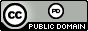시간 제한메모리 제한제출정답맞힌 사람정답 비율
2 초 512 MB75571.429%

## 문제

You have landed a lucrative contract with Amalgamated Chemical Manufacturing (ACM), to help their chemists with stoichiometry. Stoichiometry is the calculation of reactants and products in chemical reactions, based on the law of conservation of mass, which states that the total mass of the reactants equals the total mass of the products. The relations among quantities of reactants and products typically form a ratio of positive integers. If the amounts of the separate reactants are known, then the amount of the product can be calculated, and vice-versa. The relationship of reactants to products can be described using a soichiometric equation such as:

CH4 + 2O2 → CO2 + 2H2O, (1)

which can be read as: “One molecule of CH4 and two molecules of O2 yield one molecule of CO2 and two molecules of H2O.” The total number of atoms of each element on the left hand side of the stoichiometric equation must match the number of atoms of that element on right hand side. Your task is to write a program that, given an equation of the form:

_H2O + _CO2 → _O2 + _C6H12O6, (2)

will fill in the blanks to produce a balanced equation. For example, the above equation could be balanced as follows:

6H2O + 6CO2 → 6O2 + 1C6H12O6. (3)

## 입력

An equation is input in the form of a sequence of M (1 < M ≤ 20) lines, one for each molecule in the formula (e.g., H2O or CO2). Each line m (1 ≤ m ≤ M) has the following fields:

signm Nm elementm,1 countm,1 . . . elementm,Nm countm,Nm

where signm is either +1 or -1, with a sign of +1 indicating that this molecule appears on the left of the equation, and -1 indicating that it appears on the right. Nm, where 0 < Nm < 20, is the number of element/count pairs following on the line. Each elementm,n, where 1 ≤ n ≤ Nm, is an element name consisting of one or two upper or lowercase letters, and each countm,n is a positive integer, 1 ≤ countm,n ≤ 12. For example, the element/count pair “Fe 2” indicates that the molecule contains two atoms of the element Fe (iron). There will be no more than 10 unique elements in a single equation.

Note that an element may be repeated in a given line of input, as in

+1 6 C 1 H 5 C 1 O 1 O 1 H 1

which specifies that at least one molecule of CH5COOH appears on the left side of the equation. Note that CH5COOH can be written as C2H6O2.

Input ends with a line of the form

0 0

## 출력

The program must output the numbers C1, . . . , CM (0 < Ci ≤ 1 000), in order, to fill in the blanks in the equation. Each number, Cm | 1 ≤ m ≤ M, must be the minimum number for which the equation is balanced (i.e. there is no common factor that could reduce all of the Cm coefficients). You may assume that every input test case has exactly one valid solution meeting these criteria.

## 예제 입력 1

+1 2 H 2 O 1
+1 2 C 1 O 2
-1 1 O 2
-1 3 C 6 H 12 O 6
0 0


## 예제 출력 1

6 6 6 1


## 예제 입력 2

+1 5 Be 2 C 1 O 3 O 2 H 2
+1 3 Ac 1 O 1 H 1
-1 4 Be 4 O 1 Ac 6 O 6
-1 2 H 2 O 1
-1 2 C 1 O 2
0 0


## 예제 출력 2

2 6 1 5 2


## 출처• 문제를 만든 사람: Larry D. Pyeatt